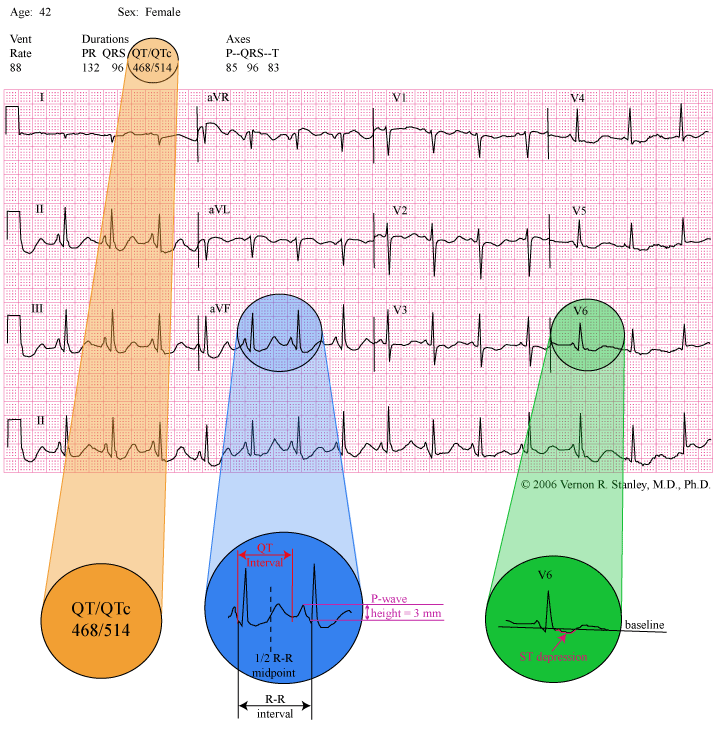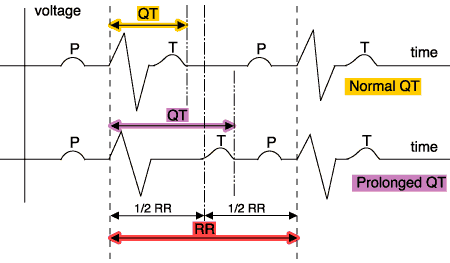# Measuring QT Prolongation & Bazett’s Equation

`From Dr. Vernon Stanley's 50 Case Study Course | Edited 7.2.20 (C Stanley, PA-C) © 2020`

With much discussion of QT Prolongation, we wanted to cover measuring the QT interval and why it’s important.

Please review the ECG below:

You are working as a paramedic and are asked to evaluate a 42-year-old female with complaints of seizure activity. Her 12-lead is as shown below and your differential diagnosis would be as follows:INTERPRETATION:

• P-wave height suggestive of right atrial enlargement.
• Diffuse ST depression and biphasic T-waves consistent with ischemia.  However, these changes are non-specific.
• Bazett’s Equation reveals a QTc in excess of 500 msec.  This represents QT prolongation and the patient is at risk for Torsades de Pointes.
• Non-specific ST-T changes

DISCUSSION:

The focus of this Case Study tracing is the QT interval.  Please note that I have magnified lead avF with labels of
R-R interval, 1/2 R-R interval and QT-interval.  The QT interval is defined as the measurement from the beginning of the QRS complex to the end of the T-wave.  Physiologically, it represents the total duration of time of the ventricular electrical activity (depolarization and repolarization).  At the end of the T-wave, the ventricles will lie dormant (in a resting state both electrically and mechanically) waiting for a stimulation from above (usually originating from the SA node), then the initiation of the QRS complex.

The importance of measuring the QT interval is that if the QT-interval is too long, the patient is at risk for a special form of ventricular tachycardia, Torsades de Pointes.  Although Torsades de Pointes is usually transient in nature, it can be sustained and even deteriorate to ventricular fibrillation and death.  It is therefore clearly important to recognize prolonged QT and avert the serious life-threatening arrhythmias of Ventricular Tachycardia and Ventricular Fibrillation if possible.

Please know that there are two distinct Categories of prolonged QT:

1.  Congenital prolonged QT-interval (prolonged QT syndrome).

2.  Acquired prolonged QT-interval.

Please be aware  that in many cases of QT prolongation, no treatment is required; but if treatment is indicated, the treatment modes are different in each category.

If ACQUIRED

– you must address the causation if possible (i.e. correct electrolyte imbalance, reverse ischemia, modify offending drug agent, etc).  Consider IV magnesium sulfate in the acute setting.

If CONGENITAL

– then consider a beta blocker and/or automatic implantable defibrillator.  Consider IV magnesium sulfate in the acute setting.

WHAT IS QT PROLONGATION AND HOW DO WE MEASURE IT?

The common thread of addressing QT prolongation of the acquired and congenital type is determining when the measured QT interval is prolonged.  What is the agreed guideline for QT prolongation?  The normal QT interval range is somewhat elusive since it is rate dependent.  As the rate increases, the QT interval decreases.  This is different from the more simple to deal with PR interval and QRS duration that are constant and not rate dependent.

There are several formulas to adjust the QT interval for rate.  For the sake of specificity and simplicity, I will present the commonly used formula by Bazett as given below.  (This equation was introduced by Bazett in the year 1920 but is still used today.)

Equation 1 (Bazett’s Equation):

QTc = QTm / square root of (R-R)

where QTc = corrected QT interval (corrected for rate)
QTm = actual measured QT interval
R-R = interval between two QRS complexes (measured in seconds)

We will agree to the following guidelines for QT prolongation:

Equation 2:

QT c > 500 msec (a range of 440 to 500 msec is a more broad guideline you will see used)

Now, let us consider the special case of rate = 60 bpm.  The R-R interval is calculated as below:

R-R = Rate (bpm) / 60 = 60 / 60 = 1 sec.

Equation 1 then becomes….

QTc = QTm / square root of (R-R) = QTm / 1 = QTm

QTc = QTm at rate of 60 bpm

For QT prolongation

We clearly see that Equation 2 becomes:

Equation 3

QTc > 500 msec

Since R-R = 1000 msec (at rate of 60 bpm)
1/2 R-R = 500 msec

Equation 3 then becomes…

QTc > 500 msec = 1/2 R-R

QTc > 1/2 R-R

Since QTc = QTm at the Rate = 60 bpm.  Equation 3 then becomes:

Equation 4

QTm > 1/2 R-R = QT prolongation

This equation holds ONLY at the rate of 60 bpm.  However, we will agree to use this as an approximation guideline if the rate is in the normal ranges (60-100 bpm).

CAUSATIONS OF PROLONGED QT-INTERVAL (see page 44 of the Rhythms Field Guide)

• Drug Effect:  amiodarone, some antibiotics, some antihistamines, some antipsychotics, phenothiazines, tricyclic antidepressants.
• Electrolyte Imbalance:  hypocalcemia, hypokalemia, hypomagnesemia.
• Miscellaneous: congenital QT prolongation, myocardial ischemia/infarction, subarachnoid hemorrhage, and others.

PEARL:

Bazett’s Equation 1 is clearly unwieldy and time consuming.  I therefore advise you to use Equation 4

(QTm  > 1/2 R-R)

as your first screening process (realizing its inaccuracy as you deviate from a rate of 60 bpm).  Although I am not a proponent of computer interpretation of the ECG, one exception is the QT interval and QTc.  The computer is programmed to calculate the QTc interval using various equations including Bazett’s or a nomogram.

Please note the magnified view of the interpretation in this Case Study tracing:

“QT / QTc = 468 / 514”

You will note that the QTc = 514 clearly exceeds the limits of QT prolongation (440 – 500 msec).

CONCLUSION:

In your systematic method of analyzing the ECG, the letter “E” of the acronym  H*E*A*R*T will prompt you to measure the QT interval.  If the rate is normal, I advise utilization of:

Equation 4

QTm > 1/2 R-R (Where QTm = measured QT)

If the QT interval crosses the midline, the QT interval is prolonged.To further refine your analysis, consult with the computer interpretation QTc.  If QTc exceeds the range (440-500 msec),

the QT interval is prolonged.

This places the patient at risk for a life-threatening arrhythmia of ventricular tachycardia (Torsades de Pointes).  This finding may need treatment in order to avoid this arrhythmia and potentially sudden death.  Other symptoms associated with this disorder might include dizziness, syncope and seizure activity.

And don’t forget that the observed prolonged QT interval might be a benign or chronic finding and indeed need no treatment at all.  It may simply be an incidental finding.  As always you must consider the clinical context and comparison with an old 12-Lead.

INTERPRETATION:

• P-wave height suggestive of right atrial enlargement.
• Diffuse ST depression and biphasic T-waves consistent with ischemia.  However, these changes are non-specific .
• Bazett’s Equation reveals a QTc in excess of 500 msec.  This represents QT prolongation and the patient is at risk for Torsades de Pointes.
• Non-specific ST-T changes

Want to see more ECG cases like this one?  Register for the 50 Case Study Course